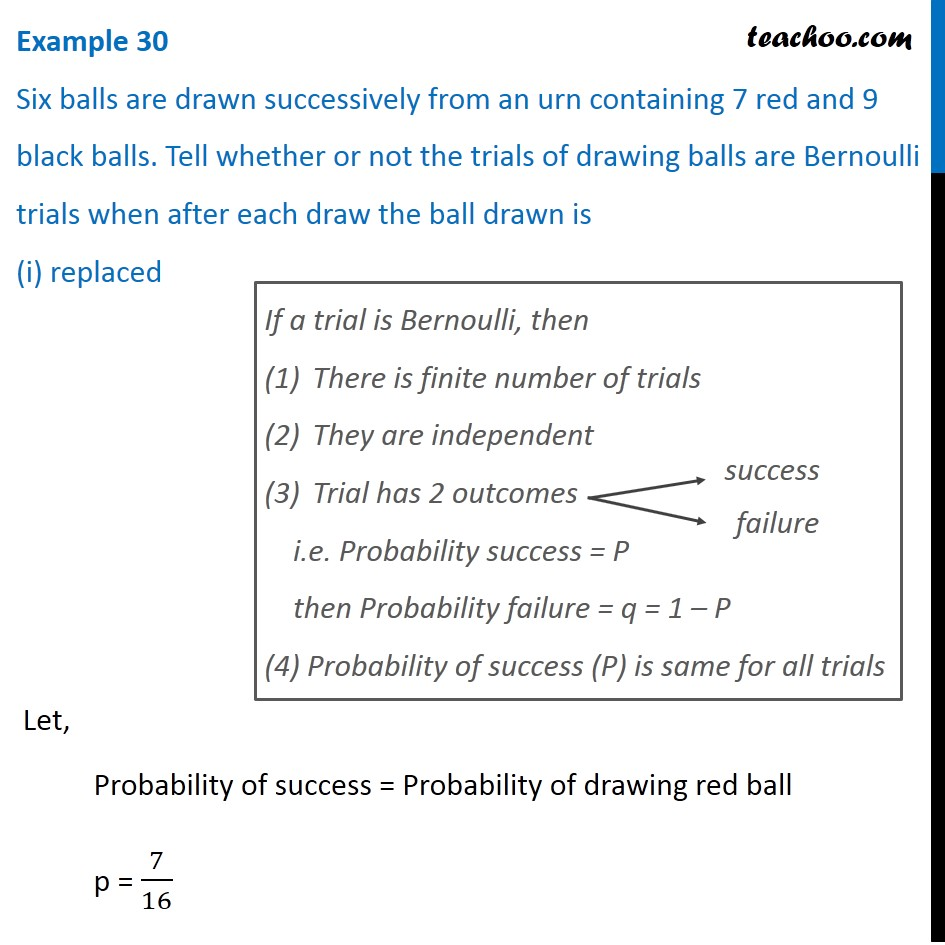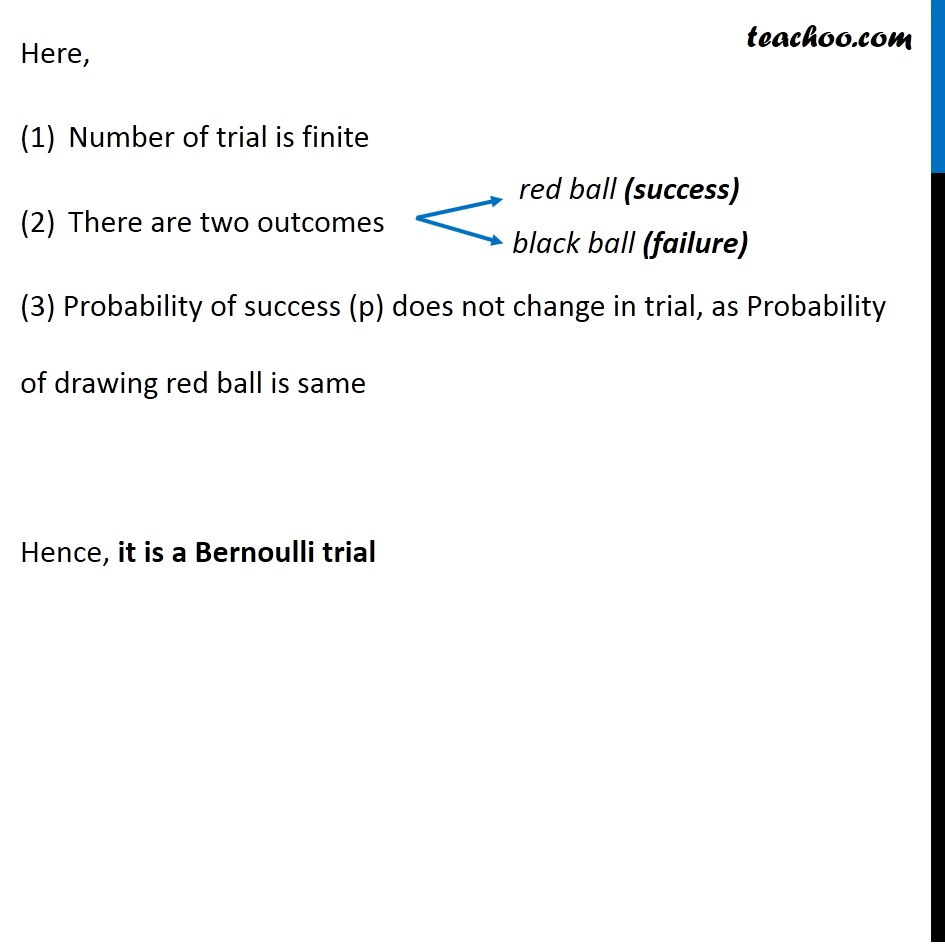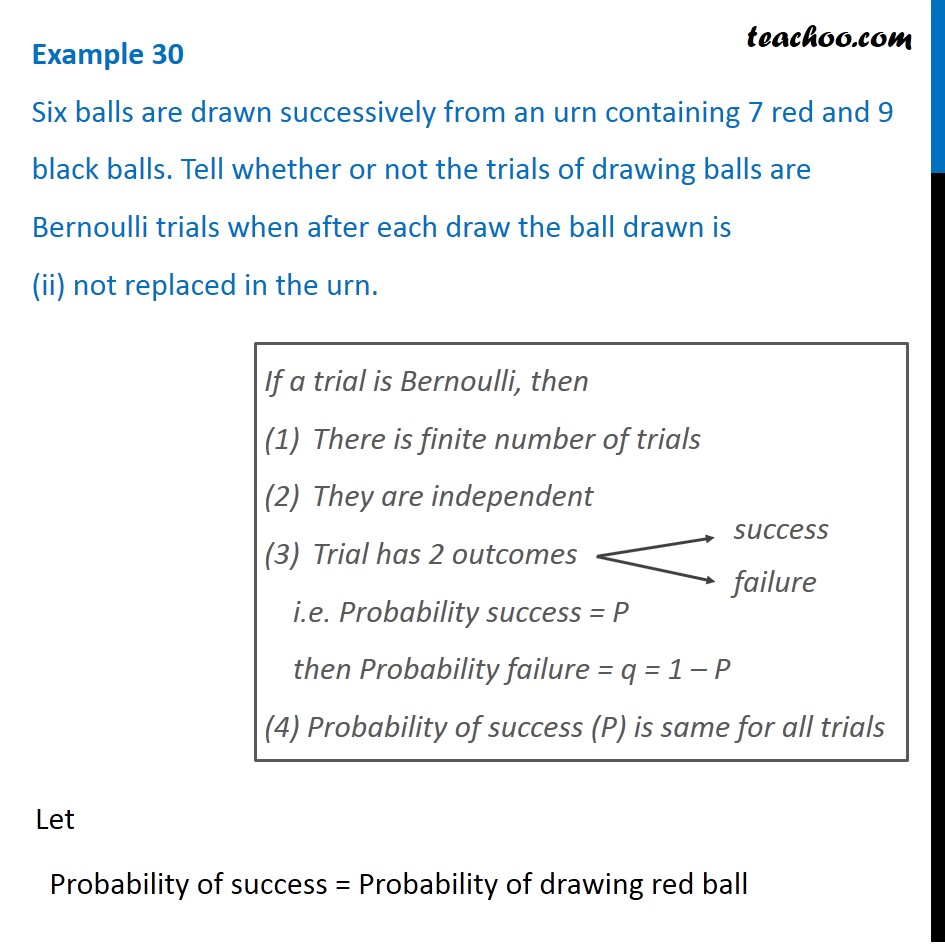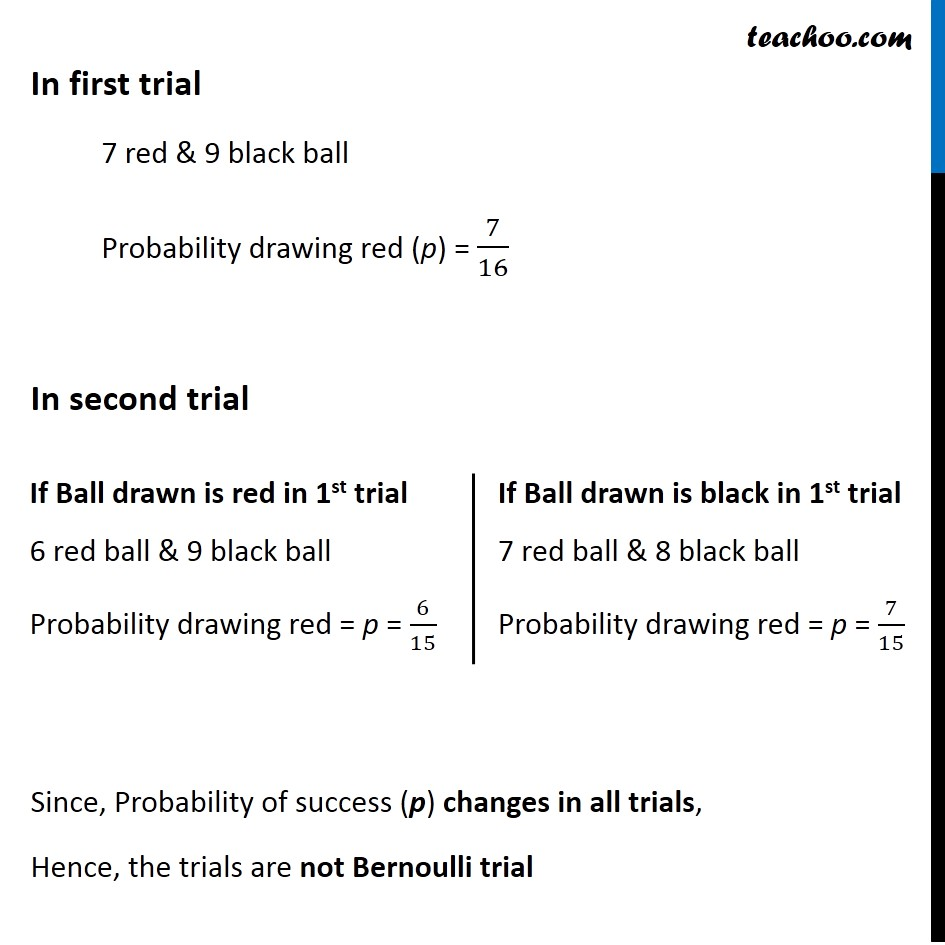Examples

Chapter 13 Class 12 Probability
Serial order wiseLearn in your speed, with individual attention - Teachoo Maths 1-on-1 Class

### Transcript

Question 9 Six balls are drawn successively from an urn containing 7 red and 9 black balls. Tell whether or not the trials of drawing balls are Bernoulli trials when after each draw the ball drawn is (i) replaced If a trial is Bernoulli, then There is finite number of trials They are independent Trial has 2 outcomes i.e. Probability success = P then Probability failure = q = 1 – P (4) Probability of success (P) is same for all trials Let, Probability of success = Probability of drawing red ball p = 7/16 Here, Number of trial is finite There are two outcomes (3) Probability of success (p) does not change in trial, as Probability of drawing red ball is same Hence, it is a Bernoulli trial Question 9 Six balls are drawn successively from an urn containing 7 red and 9 black balls. Tell whether or not the trials of drawing balls are Bernoulli trials when after each draw the ball drawn is (ii) not replaced in the urn.If a trial is Bernoulli, then There is finite number of trials They are independent Trial has 2 outcomes i.e. Probability success = P then Probability failure = q = 1 – P (4) Probability of success (P) is same for all trials Let Probability of success = Probability of drawing red ball In first trial 7 red & 9 black ball Probability drawing red (p) = 7/16 In second trial Since, Probability of success (p) changes in all trials, Hence, the trials are not Bernoulli trial If Ball drawn is red in 1st trial 6 red ball & 9 black ball Probability drawing red = p = 6/15 If Ball drawn is black in 1st trial 7 red ball & 8 black ball Probability drawing red = p = 7/15